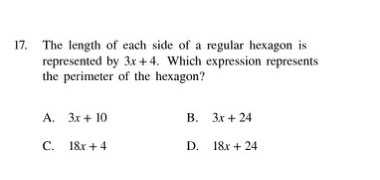### ¿Todavía tienes preguntas de matemáticas?

Pregunte a nuestros tutores expertos
Algebra
Pregunta17. The length of each side of a regular hexagon is represented by $$3 x + 4$$ . Which expression represents the perimeter of the hexagon? A. $$3 x + 10$$ B. $$3 x + 24$$

C. $$18 x + 4$$ D. $$18 x + 24$$